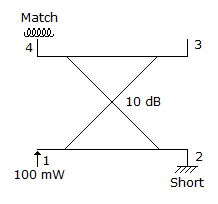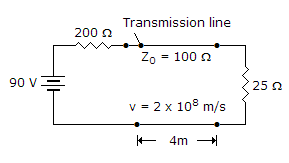# Electronics and Communication Engineering - Microwave Communication

### Exercise :: Microwave Communication - Section 5

46.

Oscillations are obtained from a reflex klystron only for combinations of anode voltage and repeller voltage that give a favourable transit time.

 A. True B. False

Answer: Option A

Explanation:

No answer description available for this question. Let us discuss.

47.

In the given figure shows a 10dB directional coupler. If power input at port 1 is 100 mW, power output at port 3 isA. 10 mW B. 15 mW C. 20 mW D. 25 mW

Answer: Option A

Explanation:

No answer description available for this question. Let us discuss.

48.

The noise figure of klystron amplifier is about

 A. 25 dB B. 3 dB C. zero D. 1 dB

Answer: Option A

Explanation:

No answer description available for this question. Let us discuss.

49.

Which one of the following is true for EM wave propagation through rectangular waveguide of cross-section 2a x a

 A. critical wavelength for TE10 mode is the same as that for TE02 mode B. critical wavelength for TE10 mode is one half of that for TE10 mode C. critical wavelength for TE10 mode is the same as that for TM10 mode D. critical wavelength for TE10 mode is double that for TE01mode

Answer: Option C

Explanation:

No answer description available for this question. Let us discuss.

50.

In the given figure the line voltage after a number of reflections will beA. 30 V B. -30 V C. 10 V D. -10 V

Answer: Option C

Explanation:

No answer description available for this question. Let us discuss.

#### Current Affairs 2021

Interview Questions and Answers